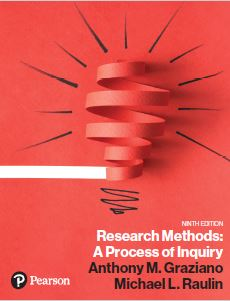﻿ Sampling Participants Using the Random Number ProgramGraziano & Raulin
Research Methods (9th edition)

## Sampling of Participants

External validity represents the extent to which our research samples accurately representing the populations to which we wish to generalize our findings. Ideally, we want to have perfectly representative samples each time, but that is impossible for two reasons. The first is that ethically we must allow potential participants to decide whether they will participate, and unless all of the participants sampled say yes, we will have some selection bias due to this ethical demand. The second is that it is very difficult to get perfectly representative samples. What we do instead is attempt to select random samples. With random samples, we know we can statistically determine the likelihood that any given sample is representative and take that into account in our inferential statistics.

A true random sample is drawn by taking all of the individuals in our population, or more likely all of the individuals in our accessible population, giving each of them a sequential number, and then selecting the number of participants needed by using random numbers. We can obtain random numbers from either a random number table or from a random number generator.

We will describe the use of both in this unit, although we will emphasize the use of a random number generator program. We have included on this Student Resource Website a instructions on how to use this program.

### Using the Random Number Table

We have included a Table of Random Numbers in Appendix B. At the beginning of Appendix B is a brief set of instructions. To select a random sample of participants from a population or accessible population, you should do the following:

1. Number every individual in the population or accessible population with a unique sequential number. For example, if you have 1217 people in your accessible population, number them from 1 to 1217.
2. Decide how many participants you will sample.
3. Select from the random number table that number of UNIQUE four-digit numbers between 1 and 1217. Each number will refer to a participant for the sample.

Selecting the set of unique numbers from the random number table usually involves several steps.

1. Select randomly a starting row. Note that the random number table in Appendix B has 200 rows. Pick any row as a starting point. You can use the random number table itself and your finger with your eyes closed to select the row if you want.
2. Since in our example we have numbered potential participants with four digit numbers, we would read across the row taking the numbers four digits at a time. The numbers are organized in blocks of five digits, but these blocks are for convenience only. The numbers in the table are single random digits from 0 to 9. If you read them in blocks of four, they represent four-digit random numbers.
3. Every time we find a four-digit number between 1 and 1217 (the number of potential participants in our example), we list that number on a separate sheet UNLESS we have already listed that number (i.e., we sample participants only once for our study).
4. We continue in this way until we have obtained the sample size we planned.

This sampling procedure works fine, but it is tedious. The Random Number Generator Program does much of the tedious work for you.

### Using the Random Number Generator Program

With the Random Number Generator Program, we can derive a list of random numbers to use for sampling in just a few seconds. We still must number all of the potential participants in our accessible population so that we will know which participants are referenced by the set of random numbers.

The instructions for using the random number program to get a list of subjects, or for other uses in research design, are available on this site.

### Stratified Random Sampling

If you want to carry out stratified random sampling using the Random Number Generator Program, you begin by creating the strata and determining how many participants you want to sample from each strata. You then treat each strata as if it were its own accessible population, and you use the same procedures described above.